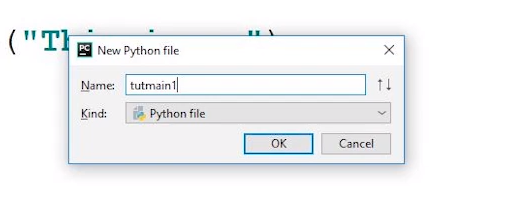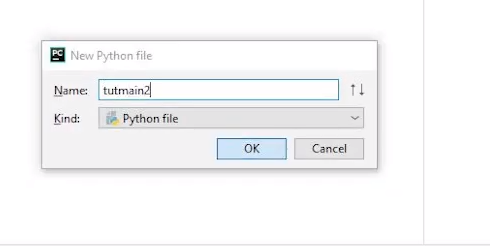### If __name__==__main__ usage & necessity

This tutorial is a little different than previous one's, as we are working with multiples files here. So let's just open our iDE and start creating our files.We are going to name our files tutmain1 and tutmain2.

Using if __name__ == “__main__” statement is one such concept that may be difficult to grasp for beginners, but once learned, it is very helpful and used quite often afterward. However, it may seem confusing at times. This article aims to provide an understanding of the behavior of the statement and further discusses how to use it. As discussed earlier, a module is just a file containing a python code with a .py extension and can be imported to other files. That's where the keyword __name__ comes in. Let’s understand __name__ first before moving onto if __name__ == “__main__.

#### What is __name__?

“A __name__ is a built-in variable that returns us the name of the module being used.”

In simple words, by using __name__, we can check whether our module is being imported or run directly.

If we run it in the same module that it is created in, then it will print “main” onto the screen; otherwise, if it is being used elsewhere, then it will print the name of its module or file it is created in.

To fully understand what __name__ is and how it is used, let us go through an example.

``````#tutmain1.py
print("__name__ in tutmain1.py is set to"+__name__)``````

#### Output:

``__name__ in tutmain1.py is set to __main__``

Let us create a new file tutmain2.py in the same directory as tutmain1.py

In this new file, let us import tutmain1.py so that we can examine the __name__ variable in tutmain2.py and let us also print the __name__ variable in tutmain2.py

``````#tutmain2.py
import tutmain1

print("__name in tutmain2.py is set to"+__name__)``````

#### Output:

``__name__ in tutmain2.py is set to tutmain1``

Let us now move further to “if __name__ == “__main__”. Working with Python files, when we import one file to another, along with the functions and variables, we also import all the print statements and other such data that we do not require. In such cases, we insert all the data of the module that we do not want others to import into the main, and thus it can only be executed by the file containing the main only.

Now we may have a certain confusion about “main”, let us clear it out first. The main is a point of the program from where the program starts its execution. Every program has its own main function. The main function can only be executed when it is being run in the same program. If the file is being imported, then it is no longer the main function because the file that is importing it has its own “main” function.

#### The syntax is :

``````if__name__=="__main__":
#Logic Statement``````

#### What are the Advantages of using if __name__ == “__main__” statement?

Following are the advantages of using if __name__ == “__main__” statement:

• Using the main in our file, we can restrict some data from exporting to other files when imported.
• We can restrict the unnecessary data, thus making the output cleaner and more readable.
• We can choose what others may import or what they may not while using our module.

To summarise the concepts discussed in this tutorial, Modules in Python has a special attribute called __name__. The value of the __name__ attribute is set to __main__ when the module is run as the main program. Otherwise, the value of __name__ is set to the name of the module. The if __name__ == “__main__ block prevents the certain code from being run when the module is imported.

#### Code file as described in the video

``````def printhar(string):
return f"Ye string harry ko de de thakur {string}"

return num1 + num2 + 5

print("aand the name is", __name__)

if __name__ == '__main__':
print(printhar("Harry1"))
print(o)

``````